# MEGR 3171 IOT Group 002 - Automated Aquarium Light

Aquarium light will turn on and off based on time of day and the amount of light in the room, sunlight or artificial light.

IntermediateFull instructions provided5 hours631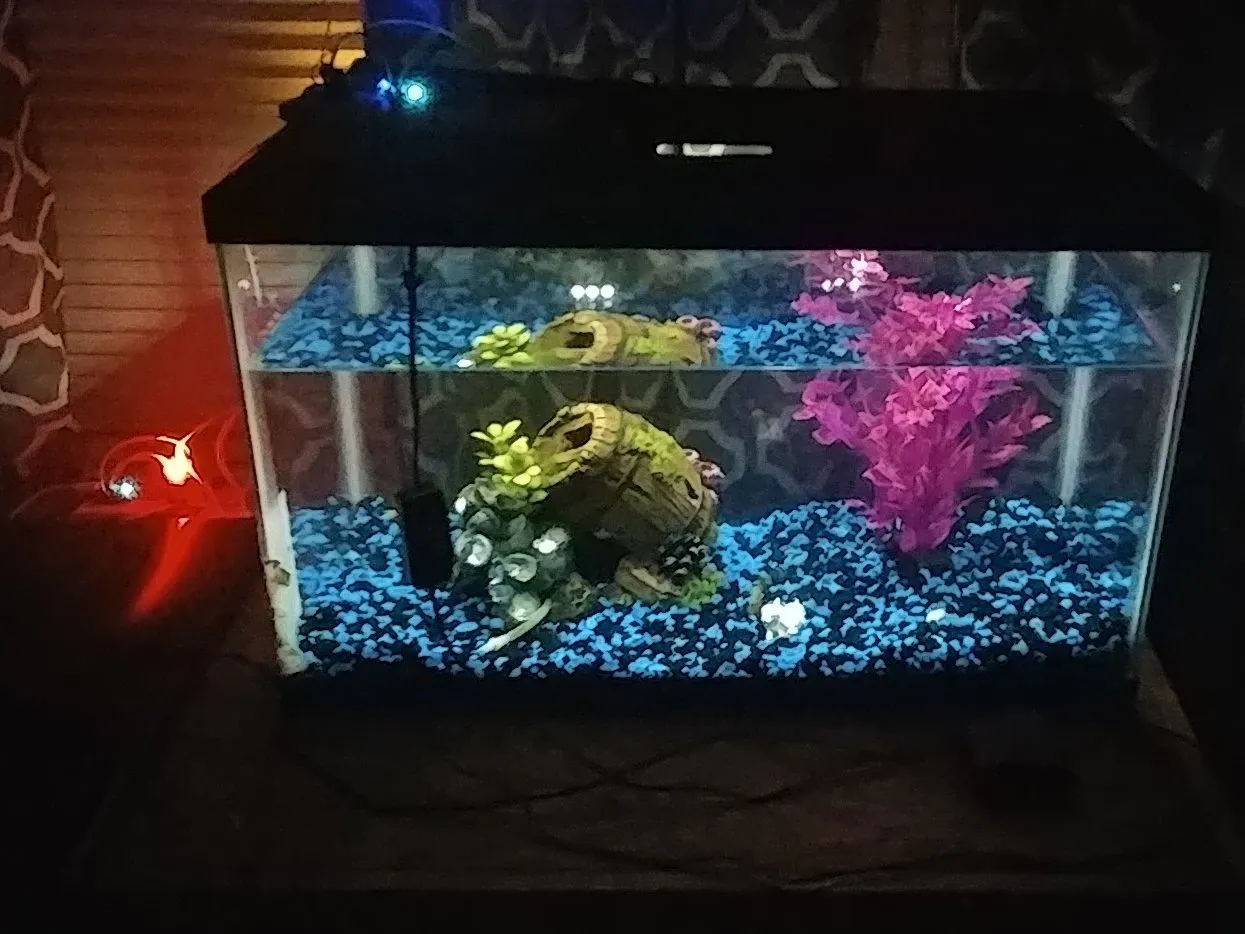## Things used in this project

### Hardware components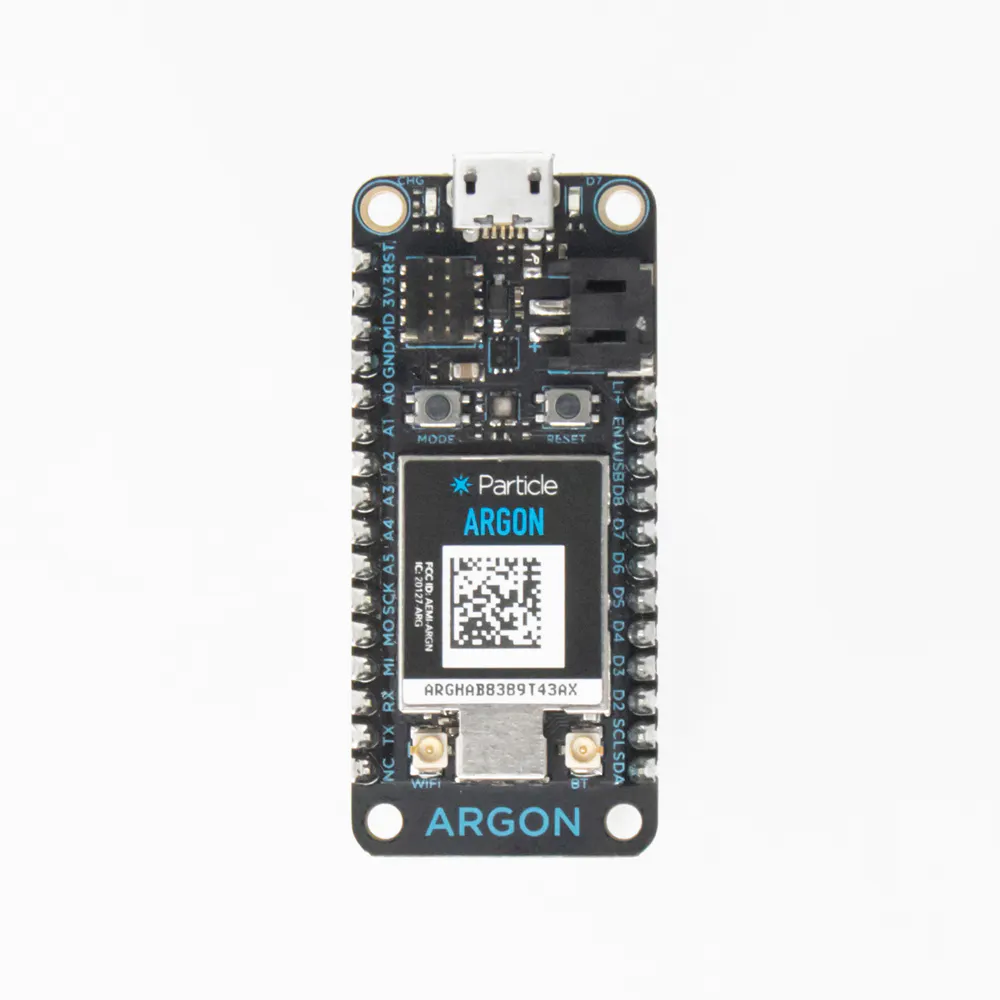Particle Argon
×2Jumper wires (generic)
×16
 Elegoo push button
×1
 Elegoo Two Color Led
×1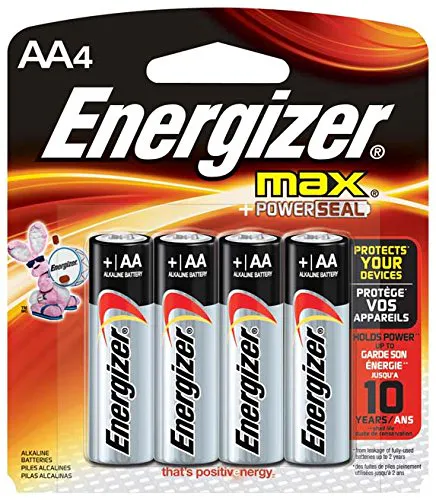AA Batteries
×4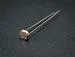Photo resistor
×1

### Software apps and online servicesParticle Build Web IDEThingSpeak API

### Hand tools and fabrication machinesTape, Electrical
 Multimeter

## Schematics

### Circuit Control Argon Circuit

Circuit diagram of the control argon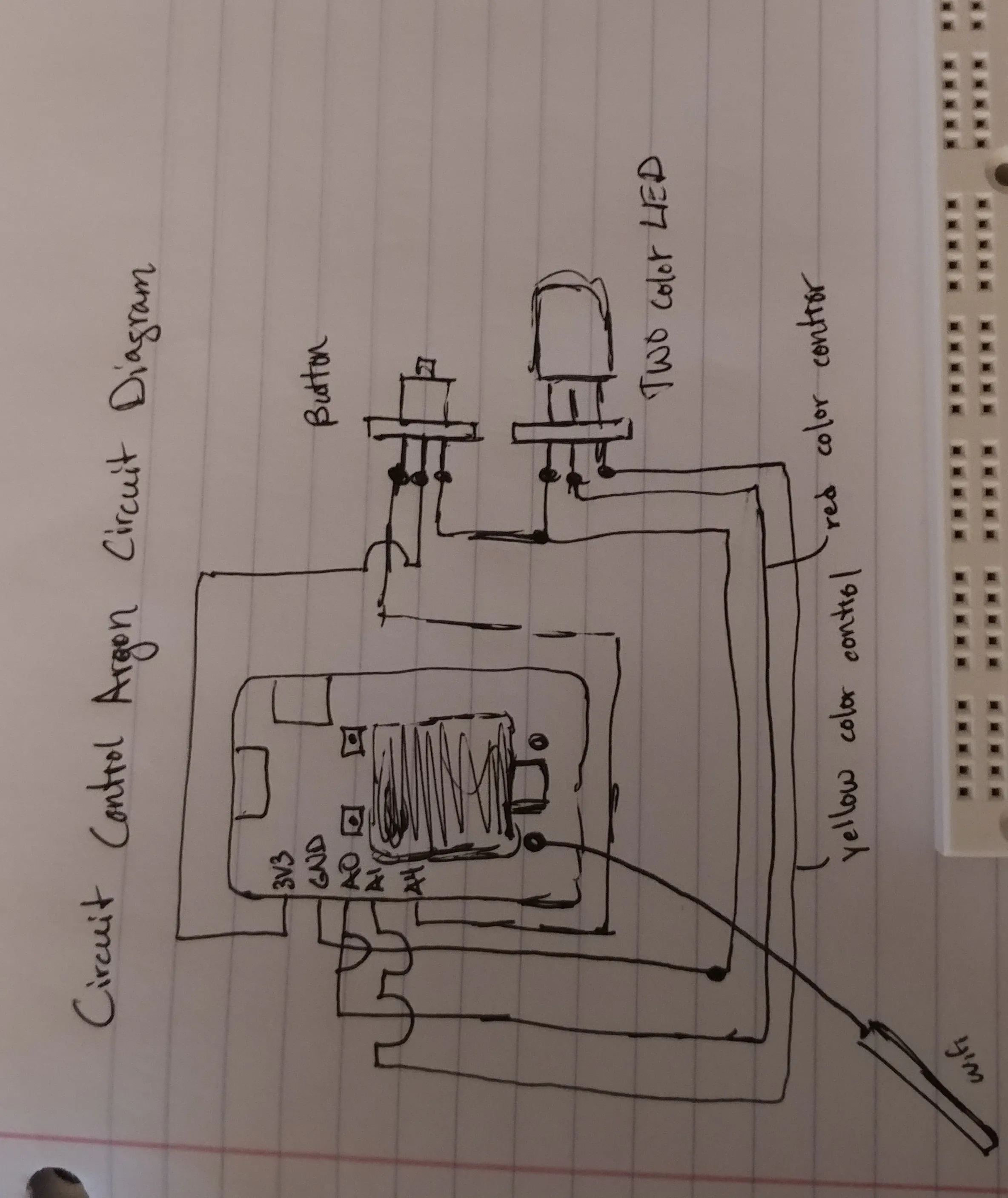### Sensing Argon Circuit

Circuit diagram of the sensing argon attached to the aquarium light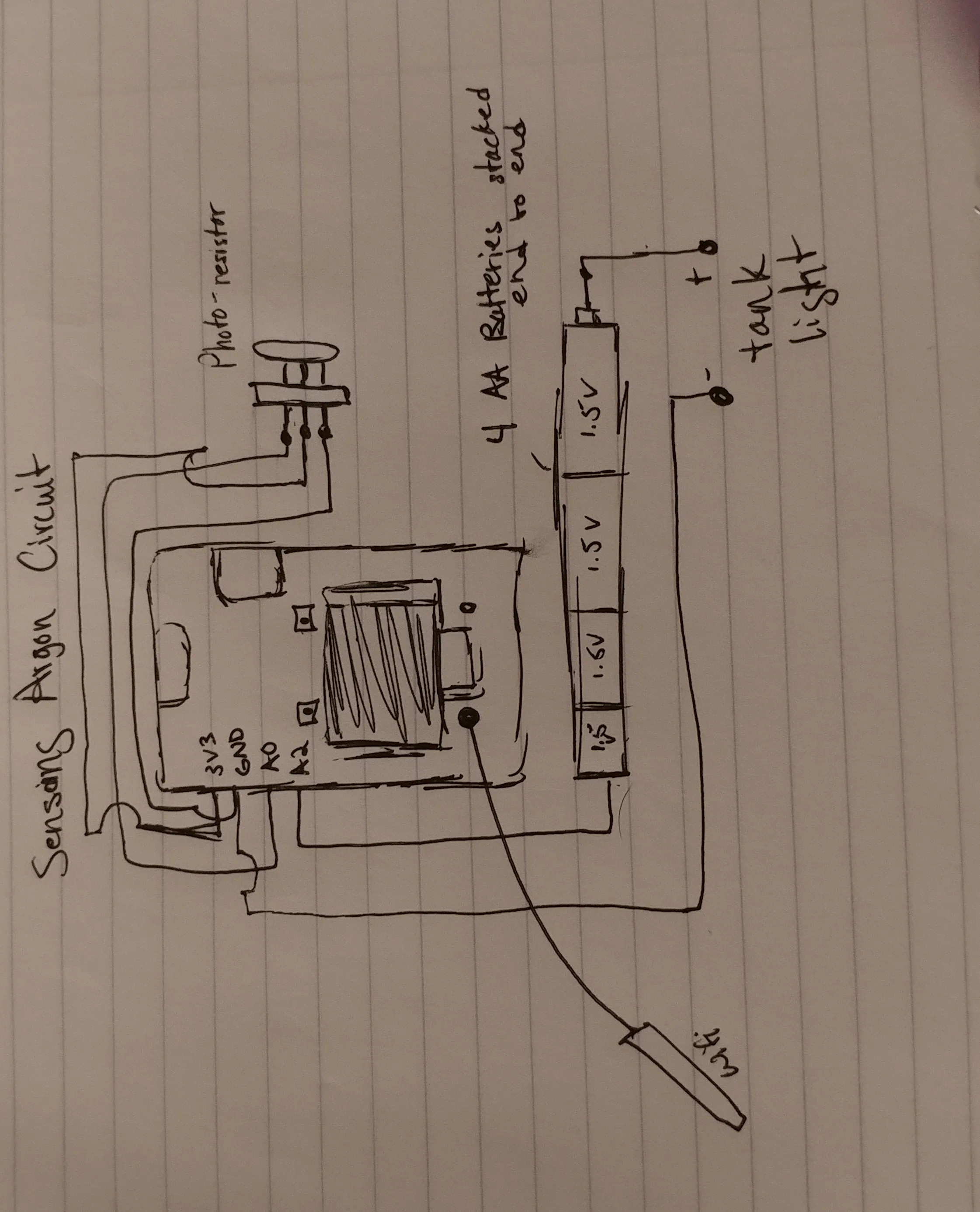## Code

### Sensing Argon Code

Processing
Code for the Sensor argon which turns the light on and off based on predetermined conditions.
```int light = A4;
int sensor = A0;
int status = D7;

int detect =0;
const int easternzone = -5;                                                                 // Constant for Eastern time zone

bool circuit_status = false;                                                                // Circuit state is off until an event is published by the other argon.

void setup(){
Serial.begin(9600);                                                                     //Hardware setup
pinMode(status, OUTPUT);                                                                //Pin Declarations
pinMode(light, OUTPUT);
pinMode(sensor, INPUT);
Time.zone(-5);                                                                          //Constant for the eastern time zone, makes inputing time constraints much easier
Particle.subscribe("circuit", circuit_stat, MY_DEVICES);                                //Subscribing to the event the other argon publishes when the button is pushed
}

void loop(){
detect = analogRead(sensor);                                                            // Setup for sensor value, states that the detect value is the analogRead value captured from the sensor
if (detect >=700  && circuit_status == true){                                          //If the value is greater than the 700, there is sufficient light in the room and the light turns off. An event is published telling the other argon the light is off.
digitalWrite(light,LOW);
Particle.publish("Argon", "light_off", PRIVATE);
String num = String(detect);                                                      //Gives thingspeak a value to graph
Particle.publish("Sensor_value", num, PRIVATE);                                     // Publishes value for thingspeak to graph, can be view in Particle Console
}
if (detect < 700 && Time.hour() >= 8 && Time.hour() <= 20 && circuit_status == true){  //If all contraints are met the aquarium light turns on and publishes event telling the other argon the light is on.
digitalWrite(light, HIGH);
Particle.publish("Argon", "light_on", PRIVATE);
String num = String(detect);                                                      //Same as above, gives thingspeak values to graph in real time
Particle.publish("Sensor_value", num, PRIVATE);
}
if (Time.hour() ==0 && circuit_status == true){                                         //Code lines 33 - 76 are all the same. They have different time values because the time function does not want to recognize < or > atfer the first statement.
digitalWrite(light, LOW);                                                           //Turns the light off at pre-determined hours, publishes event to tell the other argon the light is off. Only happens id the circuit is in the on state.
Particle.publish("Argon", "light_off", PRIVATE);
}
if (Time.hour() ==1 && circuit_status == true){
digitalWrite(light, LOW);
Particle.publish("Argon", "light_off", PRIVATE);
}
if (Time.hour() ==2 && circuit_status == true){
digitalWrite(light, LOW);
Particle.publish("Argon", "light_off", PRIVATE);
}
if (Time.hour() ==3 && circuit_status == true){
digitalWrite(light, LOW);
Particle.publish("Argon", "light_off", PRIVATE);
}
if (Time.hour() ==4 && circuit_status == true){
digitalWrite(light, LOW);
Particle.publish("Argon", "light_off", PRIVATE);
}
if (Time.hour() ==5 && circuit_status == true){
digitalWrite(light, LOW);
Particle.publish("Argon", "light_off", PRIVATE);
}
if (Time.hour() ==6 && circuit_status == true){
digitalWrite(light, LOW);
Particle.publish("Argon", "light_off", PRIVATE);
}
if (Time.hour() ==7 && circuit_status == true){
digitalWrite(light, LOW);
Particle.publish("Argon", "light_off", PRIVATE);
}
if (Time.hour() ==21 && circuit_status == true){
digitalWrite(light, LOW);
Particle.publish("Argon", "light_off", PRIVATE);
}
if (Time.hour() ==22 && circuit_status == true){
digitalWrite(light, LOW);
Particle.publish("Argon", "light_off", PRIVATE);
}
if (Time.hour() ==23 && circuit_status == true){
digitalWrite(light, LOW);
Particle.publish("Argon", "light_off", PRIVATE);
}
if (circuit_status == false){                                                               //If circuit hasn't been turned on, event is published to tell the other argon the circuit is not on.
digitalWrite(light, LOW);
Particle.publish("circuit_status", "off", PRIVATE);
delay(3000);
}
if (circuit_status == true){                                                                //Publishes event to tell the other argon that the circuit is on, this is shown by D7 light on the other argon
Particle.publish("circuit_status", "on", PRIVATE);
delay(3000);
}
}

void circuit_stat(const char *event, const char *data){                                         //Subscribed event, listens for the button to publish and then trunes the circuit on from there.
if(strcmp(data, "circuit_on")==0){
circuit_status = true;
digitalWrite(status, HIGH);

}
}
```

### Circuit Control Argon Code

Processing
Code that turns the entire circuit on, and displays the current status of the circuit.
```int light_red = A0;
int light_yellow = A1;
int status = D7;
int pushB = A4;
int bState = 0;
int sec_interval = 2;
int prev_second = 0;

void setup() {
pinMode(light_red, OUTPUT);                                          // Pin Declarations
pinMode(light_yellow, OUTPUT);
pinMode(status, OUTPUT);
pinMode(pushB, INPUT);
Particle.subscribe("Argon", status_update, MY_DEVICES);              // Subscribed to the other argon's published event
Particle.subscribe("circuit_status", Return, MY_DEVICES);            // Subscribed to the other argon's published event

}

void loop() {                                                           // loop, setup for the push button

button();

}

void button() {
if (digitalRead(pushB)==0){                                           // Senses when button was pushed
bState = 1;                                                           // button state when pressed
Particle.publish("circuit", "circuit_on", PRIVATE);                   // Publishes event for othr argon to read/use
}

}

void status_update(const char *event, const char *data){                // Function that runs when the other argon publishes "light_on" event, sets output of red led to high
if (strcmp(data, "light_on")==0){
digitalWrite(light_yellow, LOW);                              // When event is published light changes color depending on whether the circuit is on or not
digitalWrite(light_red, HIGH);                                // Turns on red light when circuit is running and the light is on
}
else if (strcmp(data, "light_off")==0){                             // Function that runs when "light_off" event is published
digitalWrite(light_yellow, HIGH);                               // Turns yellow light on to signify circuit is running but light is off
digitalWrite(light_red, LOW);
}

}
void Return(const char *event, const char *data){                       // Function that runs when "off" and "on" events are published
if(strcmp(data, "off")==0){
digitalWrite(status, HIGH);                                     // If circuit is not running the D7 light will be on
}
if(strcmp(data, "on")==0){
digitalWrite(status, LOW);                                      // If circuit is running then the D7 light will be turned off
}
}
```

## Credits

### John Hightower

1 project • 0 followers